# Arcs &Circles

Custom Workshop Pro supports ten circle and arc creation tools. From left to right along the Circle Tool palette these commands are: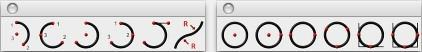Arc Tools

There are six tools for creating segmented arcs.

Two Point Arc

The Two Point Arc tool creates an arc from three points. The order of the points is the start point, the endpoint, then the CenterPoint. The Two Point Arc tool is unique in that the two endpoints are static even as the radius of the arc changes.

Using the Two Point Arc tool

• Click to set an endpoint.
• Click to set the other arc endpoint.
• Drag to set the arc’s radius and click to specify its CenterPoint.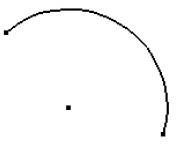Start, End, On Arc

The Start, End, On Arc tool creates an arc using three points on the arc. The first point is the starting location, the second point is the ending location, and the third point is a point that lies on the arc. The diameter is calculated based on the supplied three points.

Using the Start, End, On Arc tool

• Click the arc starting point.
• Click a point that is the arc endpoint.
• Click a point that lies along the arc.

Center Point Arc

The Center Point Arc tool creates an arc from three points. The order of the points is center point, start point, and endpoint.

Using the Center Point Arc tool

• Click the arc center point.
• Click the arc start point.
• Click a point that is scaled to the radius, terminating the arc.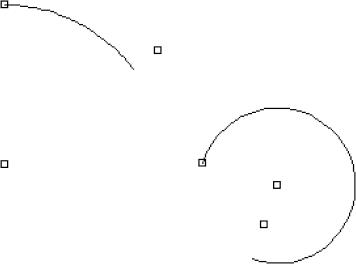Three Points Arc

The Three Points Arc tool creates an arc using three points on the arc. This tool creates an arc using first point, point on, and endpoint. The diameter is calculated based on the supplied three points.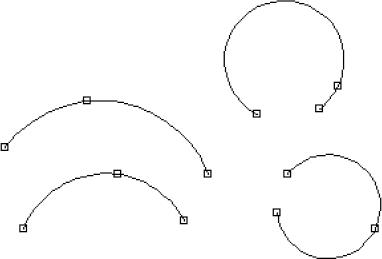Using the Three Points Arc tool

• Click the arc starting point.
• Click a point that lies along the arc.
• Click a point that is the arc endpoint.

Tangent Points Arc

The Tangent Points Arc tool creates an arc whose second point defines that tangent off of the first point. Using the Tangent Points Arc tool

• Click the arc starting point.
• Click a point that defines the starting tangent of the arc.
• Click a point that is the arc endpoint.

Arcs from Curve

The Arcs from Curve tool creates a series of arcs that follow the shape of an existing spline, ellipse or conic. Once the tool is selected, you can choose the continuity you want, on the Data Entry window.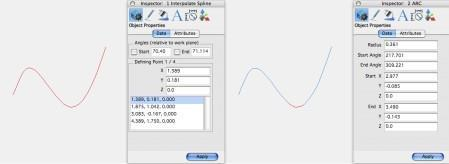Position Continuity preserves the original curve. Tangent Continuity positions each arc tangent to its counterpart. You can also enter the tolerance you want. The lower the tolerance value, the closer the arcs appears to the original shape.

Using the Arcs from Curve tool

• Click the arc starting point.
• Click a point that lies along the arc.
• Click a point that is the arc endpoint.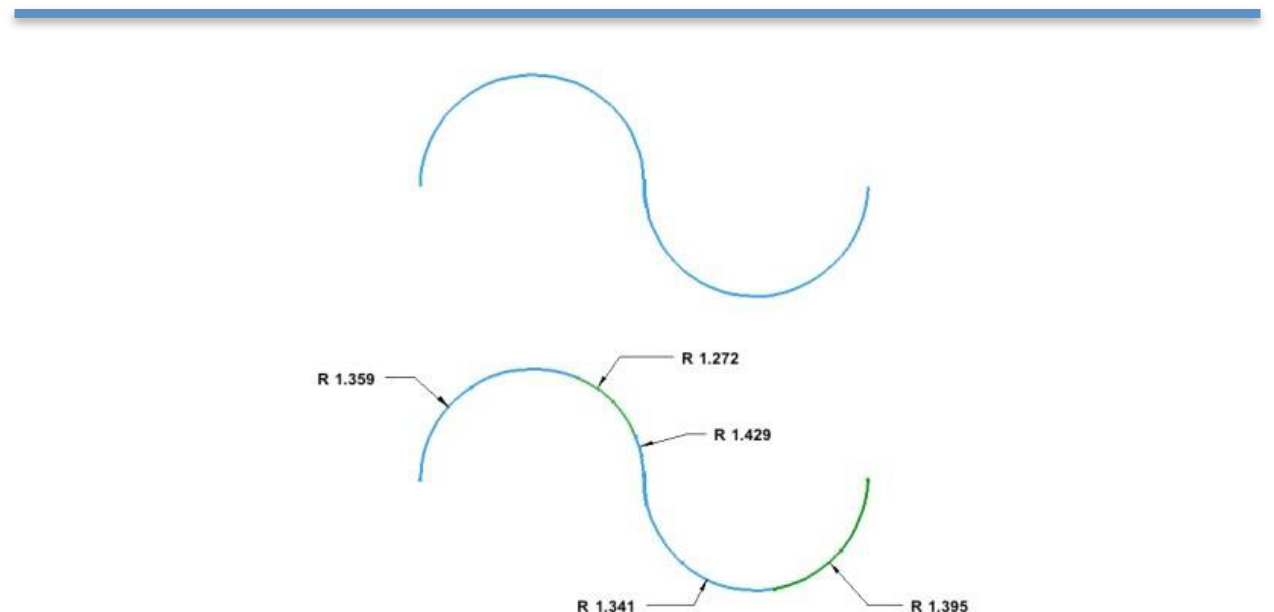Circle tools

The Circle tool palette contains six tools for creating circles.

One Point Circle

This creates a circle from one point, the centerpoint. The diameter is defined on the Data Entry Window. Using the One Point Circle tool

• Enter the diameter you want on the Data Entry Window. Be sure to press Enter to accept new values.
• Click to set the circle’s centerpoint.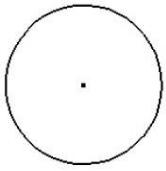Center Point Circle

Creates a circle using the first input as the circle center and the second point as a point on circle circumference.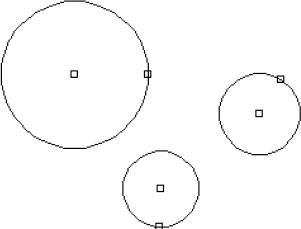Using the Center Point Circle tool

• Click the circle center point.
• Click a point that defines the circle diameter.

Opposite Point Circle

The Opposite Point Circle command creates a circle from two input points. The points lie on opposite sides of the resulting circle.

Using the Opposite Point Circle tool

• Click a point that lies on the circle circumference.
• Click a second point that lies on the opposite side of the circle.

Three Point Circle

Creates a circle from three points on the circumference.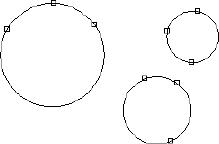Using the Three Point Circle tool

• Click the circle starting point.
• Click a point that lies along the circle.
• Click a third point that is the arc end circle.
• Hold down the Option key to make tangent to referenced curves.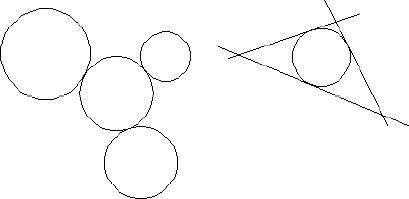Tangent Point Circle

The Tangent Point Circle tool creates a circle tangent to two curves.

Using the Tangent Point Circle tool

• Specify a diameter in the Data Entry Window.
• Click the first curve.
• Click the second curve.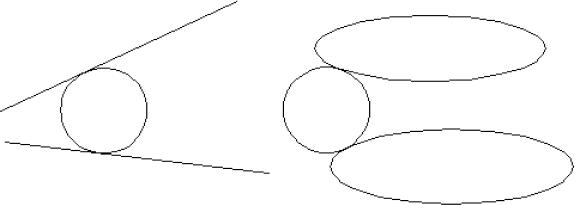Three Tangent Circle

The Three Tangent Circle tool creates a circle tangent to three curves.

Using the Three Tangent Circle tool

• Click the first curve.
• Click the second curve.
• Click the third curve.